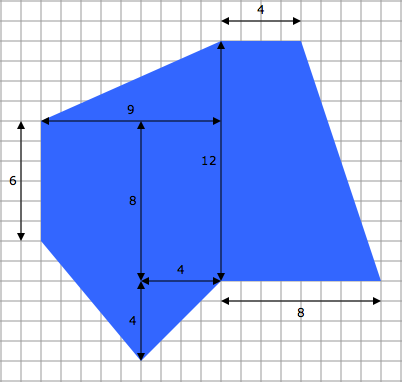We will be expanding our interactive multitouch textbook on area into a two-week sixth-grade curriculum unit for schools. This unit targets the Common Core standards 6.G.A.1 and 6.G.A.3, and includes a number of advanced features designed specifically for the classroom.

If you would like more information or are interested in helping us pilot the unit, please send us a message through our contact page.

## Common Core Standards

6.G.A.1: Find the area of right triangles, other triangles, special quadrilaterals, and polygons by composing into rectangles or decomposing into triangles and other shapes; apply these techniques in the context of solving real-world and mathematical problems.

6.G.A.3: Draw polygons in the coordinate plane given coordinates for the vertices; use coordinates to find the length of a side joining points with the same first coordinate or the same second coordinate. Apply these techniques in the context of solving real-world and mathematical problems.

## Learning Progression

The unit guides students through sequences of problems designed to help them build, apply, and extend conceptual understanding. Students begin by constructing polygons out of rectangles and right triangles and finding their area. We begin with rectangles and right triangles because finding the area of rectangles and right triangles is intuitive and makes sense.

Once that conceptual foundation has been laid, students are then able to build on it to find the area of polygons by subtracting area; deriving area formulas for general triangles, parallelograms, and trapezoids; drawing polygons in the coordinate plane; and using what they know in real-world applications.

## Targeted Interventions

The unit is designed to uncover and address common student misunderstandings. For example, some students do not understand how to determine the side lengths in a polygon when they are not given. In this unit, students use an interactive tool for drawing polygons that enables them to discover those side lengths and to develop their own understanding and rules for finding those lengths using pencil and paper.

## Complex Problem Solving

Within the first lesson of the unit, students are finding the area of complex polygons that look like this:Students quickly realize that if they truly understand how to find the area of polygons constructed using 2-3 rectangles and right triangles, then they can find the area of polygons constructed using any number of rectangles and right triangles. Finding the area of a complex polygon is not conceptually more difficult; it simply requires more care and organization. The unit comes with hundreds of problems that scale to meet the needs of all students.

## Reasoning

By guiding students through a learning progression and uncovering and addressing misunderstandings along the way, the unit enables and encourages students to reason through problems instead of trying to remember rules and procedures. The ability to reason through a problem builds confidence and deepens understanding.